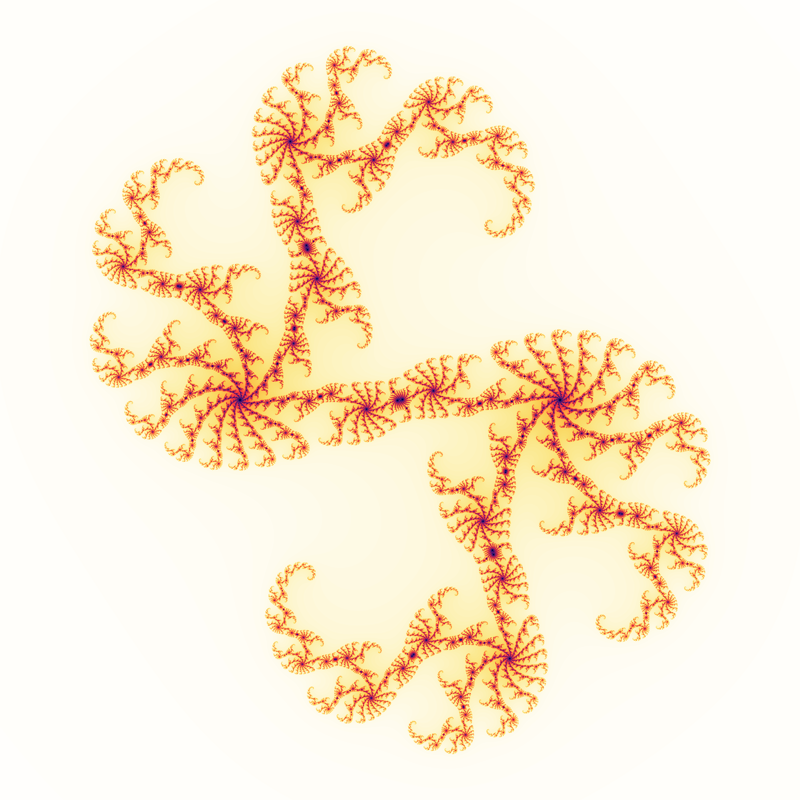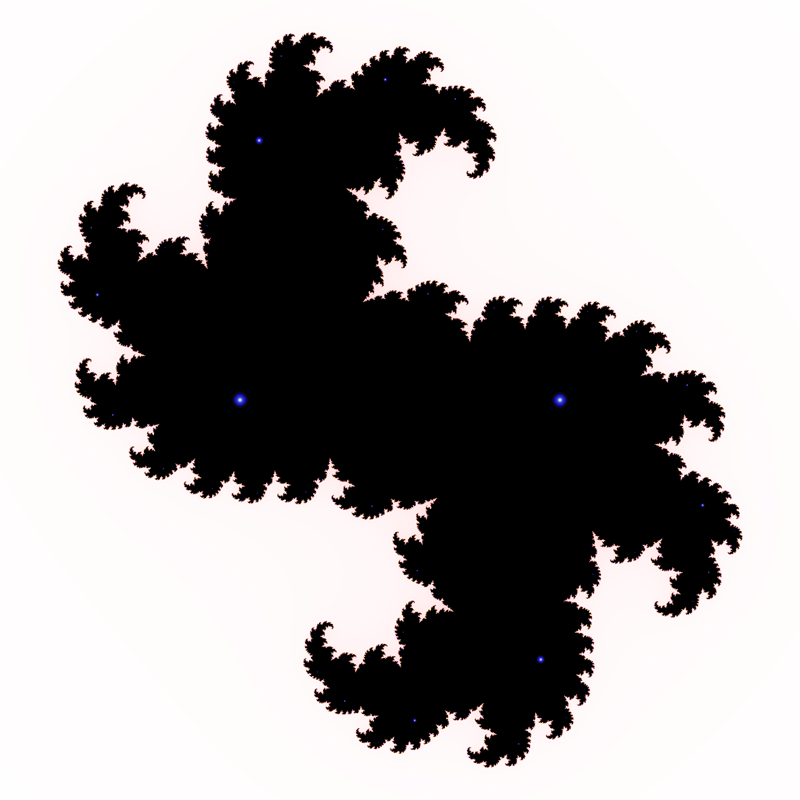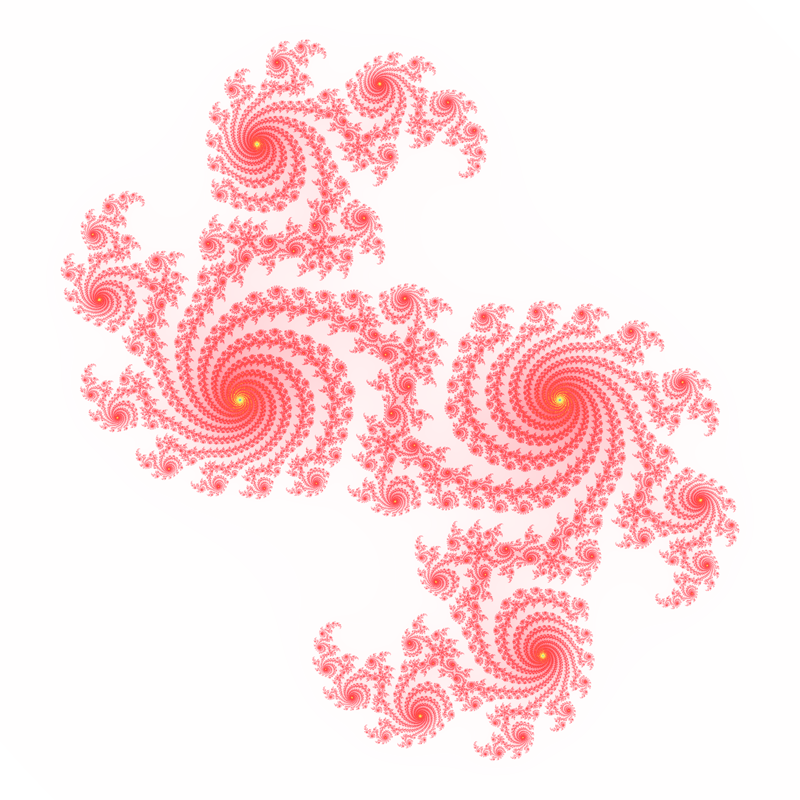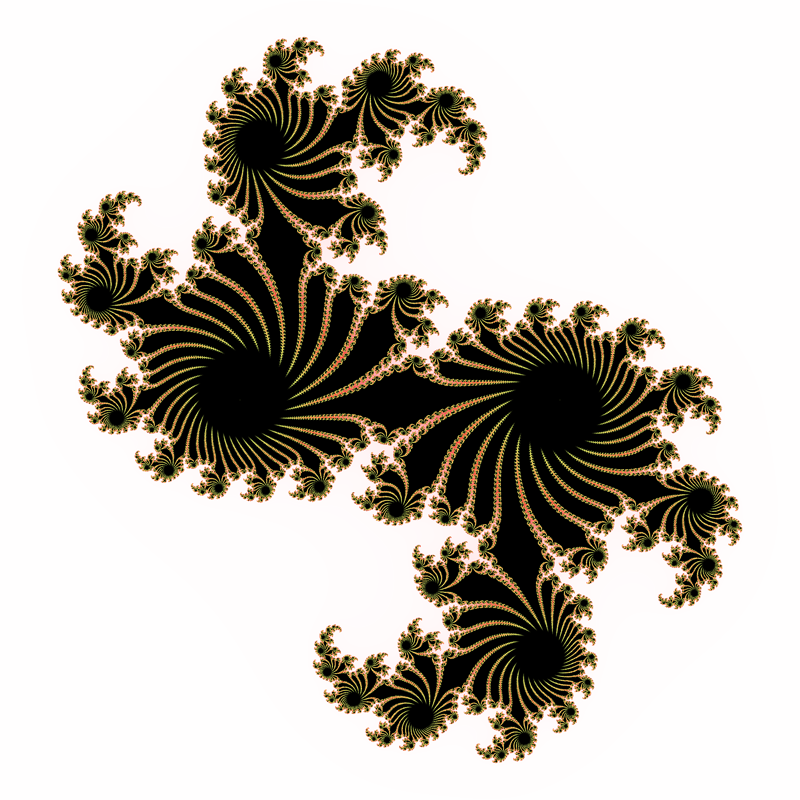# Lambda fractal

Written by Paul Bourke
December 2005

Illustration of the behavour of the series for points z0 on the complex plane.Each value of "a" gives a different fractal image, commonly used value is -(0.85 + 0.6 i).a = 0.7 + 0.7 ia = 0.7 + 0.725 ia = 0.69 + 0.725 i